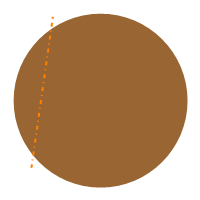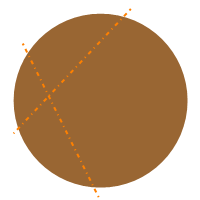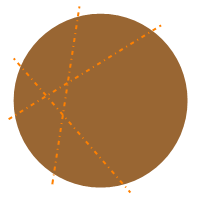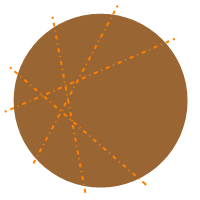# Pancake-cutting Problem

In combinatorics, the pancake-cutting problem is determining the maximum number of pieces into which a pancake can be divided by n straight cuts with a knife. This is not what we’d call a real-world problem.

Here’s one way that maximum counts of pieces for 1, 2, and 3 slices could appear.Unlike the real-world pancake-cutting problem, nobody says the pieces have to be the same size. I’m positive that people have figured out interesting arrangements of slices, such as how to make the areas of each piece as close as possible, but I haven’t been sleeping, so not really up to verifying that right now .

After staring at it for a while, we might notice that the line created by slice (n + 1) is divided into (n + 1) segments by the existing n slices: The second slice is divided into (at most) 2 segments by the existing 1 slice, the third slice is divided into (at most) 3 segments by the existing 2 slices, and (as it turns out) so on.

Throwing in the “(at most)” parts since we’re going for the maximum number of pieces. For a minimum number of pieces, we could make a bunch of parallel slices, where slice n results in (n + 1) pieces. Somewhere between that and the maximum, every slice could go through the center of the circle, where slice n results in 2n pieces, per traditional cake cutting.

By the way, how do you pronounce the ordinal for (n + 1)? “n + 1th”? “n + first”? “n + oneth”?

Once we notice that, as in the figures above, we might notice that each segment of a new slice divides one existing region into two pieces.

• Slice 1 divides the 1 region (the whole pancake) into 2 pieces.
• Slice 2 divides 2 regions into 4 pieces.
• Slice 3 divides 3 existing regions into 6 pieces, leaving 1 region untouched, for a total of 7 pieces.
• Slice 4 divides 4 existing regions into 8 pieces, leaving 3 regions untouched, for a total of 11 pieces.Let’s use the name f(n) for the number of pieces after n cuts have been made. Then cut (n + 1) results in 2(n + 1) pieces created by dividing (n + 1) of the existing regions, plus the existing f(n)−(n + 1) untouched regions.

This simplifies to the recurrence relation f(n + 1) = f(n) + (n + 1) with initial condition f(0)=1. Solving the recurrence, we see that the number of pancake pieces after n cuts is (n2 + n + 2)/2.

See Martin Gardner, Martin Gardner’s New Mathematical Diversions from Scientific American, pp. 235–239, 1961, and Fred S. Roberts, Applied Combinatorics, pp. 198–200, 1984.

Figures created with Mathematica 10.1.